﻿ IDAPython：让你的生活更美好（二）-合天网安新闻 ﻿

IDAPython：让你的生活更美好（二）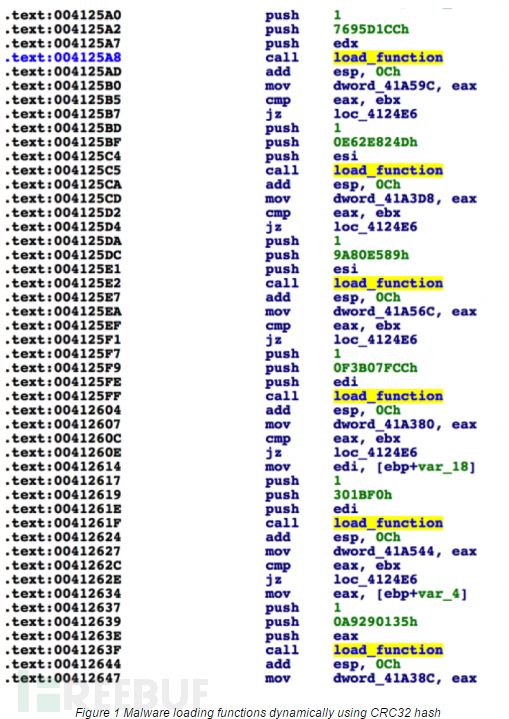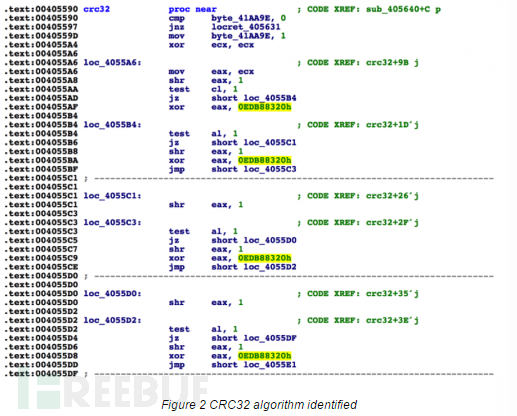```def get_functions(dll_path):
pe = pefile.PE(dll_path)
if ((not hasattr(pe, 'DIRECTORY_ENTRY_EXPORT')) or (pe.DIRECTORY_ENTRY_EXPORT is None)):
print "[*] No exports for %s" % dll_path
return []
else:
expname = []
for exp in pe.DIRECTORY_ENTRY_EXPORT.symbols:
if exp.name:
expname.append(exp.name)
return expname```

```def calc_crc32(string):
return int(binascii.crc32(string) & 0xFFFFFFFF)```

`HASH => NAME`

https://github.com/pan-unit42/public_tools/blob/master/ida_scripts/gen_function_json.py

```for k,v in json_data.iteritems():
json_data[int(k)] = json_data.pop(k)```

http://www.cprogramming.com/tutorial/enum.html）

```enumeration = GetEnum("crc32_functions")
if enumeration == 0xFFFFFFFF: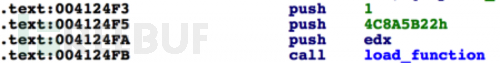```for x in XrefsTo(load_function_address, flags=0):
push_count = 0
push_count += 1
if push_count == 2:
if data in json_data:
name = json_data[data]```

`AddConstEx(enumeration, str(name), int(data), -1)`

```def get_enum(constant):
all_enums = GetEnumQty()
for i in range(0, all_enums):
enum_id = GetnEnum(i)
enum_constant = GetFirstConst(enum_id, -1)
name = GetConstName(GetConstEx(enum_id, enum_constant, 0, -1))
if int(enum_constant) == constant: return [name, enum_id]
while True:
enum_constant = GetNextConst(enum_id, enum_constant, -1)
name = GetConstName(GetConstEx(enum_id, enum_constant, 0, -1))
if enum_constant == 0xFFFFFFFF:
break
if int(enum_constant) == constant: return [name, enum_id]
return None

enum_data = get_enum(constant)
if enum_data:
name, enum_id = enum_data
return True
else:
return False```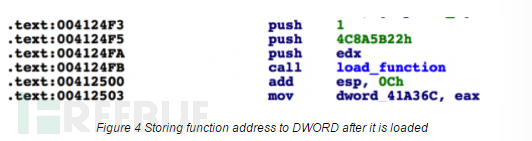```address = current_address
MakeName(operand_value, str("d_"+name))```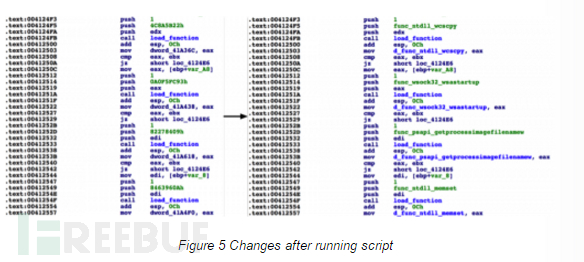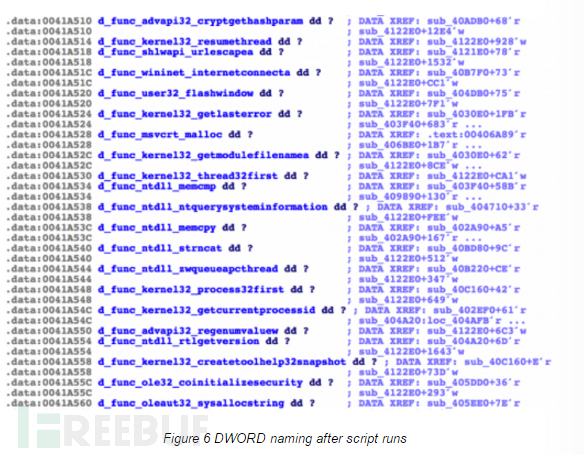﻿